### 14.2.1.1 Reachable set

Assume temporarily that there are no obstacles: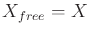. Let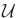be the set of all permissible action trajectories on the time interval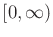. From each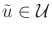, a state trajectory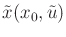is defined using (14.1).Which states inare visited by these trajectories? It may be possible that all ofis visited, but in general some states may not be reachable due to differential constraints.

Let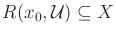denote the reachable set from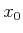, which is the set of all states that are visited by any trajectories that start atand are obtained from someby integration. This can be expressed formally as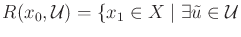and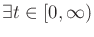such that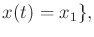(14.4)

in which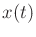is given by (14.1) and requires that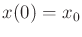.

The following example illustrates some simple cases.

Example 14..2 (Reachable Sets for Simple Inequality Constraints)   Suppose that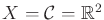, and recall some of the simple constraints from Section 13.1.1. Let a point in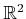be denoted as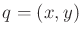. Let the state transition equation be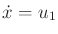and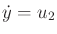, in which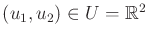.

Several constraints will now be imposed on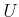, to define different possible action spaces. Suppose it is required that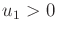(this was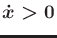in Section 13.1.1). The reachable set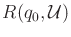from any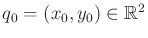is an open half-plane that is defined by the set of all points to the right of the vertical line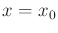. In the case of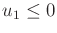, thenis a closed half-plane to the left of the same vertical line. Ifis defined as the set of all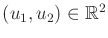such thatand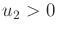, then the reachable set from any point is a quadrant.

For the constraint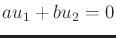, the reachable set from any point is a line inwith normal vector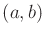. The location of the line depends on the particular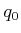. Thus, a family of parallel lines is obtained by considering reachable states from different initial states. This is an example of a foliation in differential geometry, and the lines are called leaves .

In the case of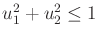, the reachable set from any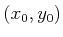is. Thus, any state can reach any other state.So far the obstacle region has not been considered. Let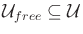denote the set of all action trajectories that produce state trajectories that map into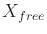. In other words,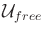is obtained by removing fromall action trajectories that cause entry into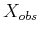for some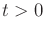. The reachable set that takes the obstacle region into account is denoted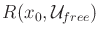, which replacesbyin (14.4). This assumes that for the trajectories in, the termination action can be applied to avoid inevitable collisions due to momentum. A smaller reachable set could have been defined that eliminates trajectories for which collision inevitably occurs without applying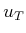.

The completeness of an algorithm can be expressed in terms of reachable sets. For any given pair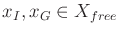, a complete algorithm must report a solution action trajectory if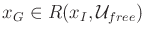, or report failure otherwise. Completeness is too difficult to achieve, except for very limited cases [171,747]; therefore, sampling-based notions of completeness are more valuable.

Steven M LaValle 2020-08-14